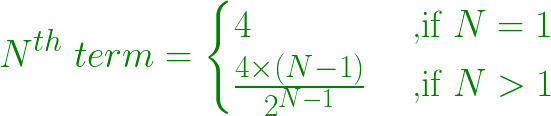Skip to content
Related Articles
Find Nth term of the series 4, 2, 2, 3, 6, …
• Last Updated : 18 Mar, 2021

Given a number N, the task is to find the N-th term in series 4, 2, 2, 3, 6, …
Example:

```Input: N = 2
Output: 2

Input: N = 5
Output: 6```

Approach:

• Nth number of the series is obtained by
1. Multiplying the previous number with the position of the previous number itself.
2. Divide the obtained number by 2.
• Since the starting number of the series is 4

```1st term = 4
2nd term = (4 * 1) / 2 = 2
3rd term = (2 * 2) / 2 = 2
4th term = (2 * 3) / 2 = 3
5th term = (3 * 4) / 2 = 6
And, so on....```
•
• In general, Nth number is obtained by formula:•

Below is the implementation of the above approach:

## C++

 `// C++ program to find Nth term``// of the series 4, 2, 2, 3, 6, ...` `#include ``using` `namespace` `std;` `// Function to find Nth term``int` `nthTerm(``int` `N)``{``    ``int` `nth = 0, first_term = 4;` `    ``int` `pi = 1, po = 1;``    ``int` `n = N;``    ``while` `(n > 1) {``        ``pi *= n - 1;``        ``n--;``        ``po *= 2;``    ``}` `    ``// Nth term``    ``nth = (first_term * pi) / po;` `    ``return` `nth;``}` `// Driver code``int` `main()``{``    ``int` `N = 5;` `    ``cout << nthTerm(N) << endl;` `    ``return` `0;``}`

## Java

 `// Java program to find Nth term``// of the series 4, 2, 2, 3, 6, ...``class` `GFG``{` `// Function to find Nth term``static` `int` `nthTerm(``int` `N)``{``    ``int` `nth = ``0``, first_term = ``4``;` `    ``int` `pi = ``1``, po = ``1``;``    ``int` `n = N;``    ``while` `(n > ``1``)``    ``{``        ``pi *= n - ``1``;``        ``n--;``        ``po *= ``2``;``    ``}` `    ``// Nth term``    ``nth = (first_term * pi) / po;` `    ``return` `nth;``}` `// Driver code``public` `static` `void` `main(String[] args)``{``    ``int` `N = ``5``;` `    ``System.out.print(nthTerm(N) +``"\n"``);``}``}` `// This code is contributed by Rajput-Ji`

## Python3

 `# Python3 program to find Nth term``# of the series 4, 2, 2, 3, 6, ...` `# Function to find Nth term``def` `nthTerm(N) :` `    ``nth ``=` `0``; first_term ``=` `4``;` `    ``pi ``=` `1``; po ``=` `1``;``    ``n ``=` `N;``    ``while` `(n > ``1``) :``        ``pi ``*``=` `n ``-` `1``;``        ``n ``-``=` `1``;``        ``po ``*``=` `2``;` `    ``# Nth term``    ``nth ``=` `(first_term ``*` `pi) ``/``/` `po;` `    ``return` `nth;` `# Driver code``if` `__name__ ``=``=` `"__main__"` `:``    ``N ``=` `5``;``    ``print``(nthTerm(N)) ;` `# This code is contributed by AnkitRai01`

## C#

 `// C# program to find Nth term``// of the series 4, 2, 2, 3, 6, ...``using` `System;` `class` `GFG``{` `// Function to find Nth term``static` `int` `nthTerm(``int` `N)``{``    ``int` `nth = 0, first_term = 4;` `    ``int` `pi = 1, po = 1;``    ``int` `n = N;``    ``while` `(n > 1)``    ``{``        ``pi *= n - 1;``        ``n--;``        ``po *= 2;``    ``}` `    ``// Nth term``    ``nth = (first_term * pi) / po;` `    ``return` `nth;``}` `// Driver code``public` `static` `void` `Main(String[] args)``{``    ``int` `N = 5;` `    ``Console.Write(nthTerm(N) +``"\n"``);``}``}` `// This code is contributed by PrinciRaj1992`

## Javascript

 ``
Output:
`6`

Attention reader! Don’t stop learning now. Get hold of all the important DSA concepts with the DSA Self Paced Course at a student-friendly price and become industry ready.  To complete your preparation from learning a language to DS Algo and many more,  please refer Complete Interview Preparation Course.

In case you wish to attend live classes with industry experts, please refer DSA Live Classes

My Personal Notes arrow_drop_up